#### Amped_86

Joined Jun 28, 2018
26
I'm currently learning about electromagnetic induction. I've come across a part of it that I'm either wrong about or don't understand fully.This graph is from the transformers chapter in the education section of AAC. At the very start of the graph, if the voltage waveform is in the positive (upper) part of the graph and the current and magnetic flux are in the negative (lower) part of the graph, does this mean that these waveforms are of opposite polarity?

#### BobTPH

Joined Jun 5, 2013
6,508
No, it means they are out of phase by 90 degrees.

Reversed polarity would have one at the positive peak when the other is at the negative peak.

Bob

#### Amped_86

Joined Jun 28, 2018
26
No, it means they are out of phase by 90 degrees.

Reversed polarity would have one at the positive peak when the other is at the negative peak.

BobThis graph is from the reactance and Impedance - Inductance chapter in AAC's education section. In this graph you can see a positive sign in the upper part and a negative sign in the lower part. You have to refer to the end of this graph to make it match with the first one. Excluding the power waveform, if the voltage waveform and the current waveform are not of opposite polarity (at the end of the cycle), what do the positive and negative signs mean then? I understand that the AC waveforms above don't have 'polarity' in the traditional sense that we see with DC circuits. But if I went to the end of the above graph where the voltage waveform is in the positive part of the graph and the current in the negative part and took an instantaneous snapshot, could I say that the voltage and current waveforms are of opposite polarity (opposing each other), albeit not completely (exactly 180 degrees) but only partially as you indicated (90 degrees)?

#### MrSoftware

Joined Oct 29, 2013
2,117
In regards to your first post; they've taken a snapshot in time of a system that is already running in a stable state. The graph is not starting at time 0. A coil has inductance so the current will lag behind the voltage.

•Kjeldgaard

#### BR-549

Joined Sep 22, 2013
4,928
For a portion of time.....the voltage and current are in opposite polarity. But we don't call it that. When we talk of voltage and the current caused by THAT voltage......we call it phase. And it's not called opposite....it's called leading or lagging.

Joined Jan 15, 2015
7,192
I'm getting pretty old. When I grew up we were Po Folks and didn't even have a refrigerator but we had an ice box. Every couple of days a guy came around in a truck and dropped off a big block of ice. I remember his name, he was ELI the ICE Man and he really did live in a cave.Ron

•nsaspook

#### djsfantasi

Joined Apr 11, 2010
8,884
I'm getting pretty old. When I grew up we were Po Folks and didn't even have a refrigerator but we had an ice box. Every couple of days a guy came around in a truck and dropped off a big block of ice. I remember his name, he was ELI the ICE Man and he really did live in a cave.Ron
We had a ‘fridge, but camped a lot. The campground had an old guy with a truck full of ice blocks. The only insulation was an old canvas tarp over the ice. We kids would run after him and he’d chip of bits for us to suck on.

Joined Jan 15, 2015
7,192
We had a ‘fridge, but camped a lot. The campground had an old guy with a truck full of ice blocks. The only insulation was an old canvas tarp over the ice. We kids would run after him and he’d chip of bits for us to suck on.
Yeah, a canvas tarp over the ice. We did have an old Philco but my dad did have an ice box and the ice man. Blocks of ice under a tarp. Ice Houses would cut large blocks of ice from frozen lakes and most of it would last through the spring and summer.

Today I check into a motel, take my ice bucket to the ice machine, get my ice and pour a scotch. Life is good. ELI the ICE Man was my gouge in school. I have no clue how it originated but it was years before as the then old guys learned it many decades before I ever herd of it.

Ron

#### Tonyr1084

Joined Sep 24, 2015
7,353
To avoid confusion - look at the second sine wave of the voltage. As you can see at this point the system is running (whatever it's doing). The preceding waveform reveals why the voltage (at zero and rising) has the current lagging behind (90˚). Next, focus on the peak of the voltage. At that point there is no change in the voltage and all current comes to zero (neither positive or negative). As the voltage begins to change (downward sweep) the current begins to rise in the (opposite) direction. Current in an inductor rises when the voltage is changing. When the voltage is stable (peak positive or peak negative) there is no change in said voltage. No change means zero current.

Capacitors are just the opposite. When voltage BEGINS to go up current into a cap is at max. As the voltage reaches peak the current in the cap is zero. This is known as "Leading" current. In other words (and as simple as I know how to explain it) an empty capacitor acts like a short. When power is applied - that inrush of current is at max until the capacitor reaches full charge. Between max current and max voltage is a sine wave similar to the one you've shown.

But sticking with inductors, they don't develop max current until the voltage is changing and crossing zero threshold.

Someone correct me if I'm wrong.

#### BobTPH

Joined Jun 5, 2013
6,508
You are wrong. The current in an inductor increases as long as there is a voltage across it. The voltage does not have to be changing. Keeping a comstat voltage on an inductor will cause the current to continue rising until something breaks or it is limited by the internal resistance.

When the input is a sin wave, the current increases during the entire positive half of the wave, reaching a max when the voltage hits zero. The the current starts decreasing and finally reversing during the negative half of the cycle.

Bob

#### Tonyr1084

Joined Sep 24, 2015
7,353
@BobTPH Yeah - I was thinking purely of AC. You're right, DC would produce a constant current. If I recall, AC does not pass through an inductor (a choke is what it's called I believe) Thanks for covering my error.

#### Amped_86

Joined Jun 28, 2018
26
Thank you for the replies. The ELI the ICE man pneumonic/acronym is great. I’m still confused about a couple of things.

If we start at the beginning of the graph again where the voltage is at zero, at that point in time the current and is at a minimum. The voltage and current are also of opposite polarities from each other. This is denoted by the voltage being in the positive part of the graph and the current being in the negative part of the graph. Moving along, the current’s rate of change begins to increase. The slope of the current waveform is getting steeper and steeper. However, the magnitude of the current is actually decreasing. The current is of a negative polarity, but its magnitude is decreasing and getting closer to zero. Inductors oppose changes in current and will drop a voltage across the coils of the inductor that opposes this change. Therefore, the voltage waveform will begin to increase as the rate of change of the current begins to increase. But the inductor wants to oppose this rate of change so it will drop a voltage that opposes the changing current. I reasoned that this is why the induced voltage is 90 degrees ahead of the current, and why the voltage waveform is of opposite polarity or in the positive part of the graph when the current is in the negative part of the graph. For a long time, this was confusing to me because it looked like the current was decreasing. And it is in magnitude, but the rate of change of current or the steepness of the slope is actually increasing as it gets closer and closer to a magnitude of zero. The induced voltage is the derivative of the current. The voltage is now at a maximum in the positive part of the graph, and the current is at zero because this is the steepest part of the current waveform’s slope. Is this correct?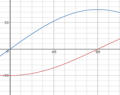Induced voltage is the blue wave form and current is the red waveform

At this point my intuition and reasoning begin to break down. But this is what I’ve come up with so far. At the horizontal axis, the current switches polarity. I’ve always visualized current as moving in a particular direction. Is this correct? Does this mean that at the horizontal axis, the current literally switches directions? It’s hard to picture the voltage opposing anything when the current is zero in magnitude. Moving on from this point, as the current passes the horizontal axis and begins to increase in positive magnitude, its rate of change actually begins to decrease. Again, we have to consider the derivative of the current. The induced voltage is still the derivative of the current, and its still trying to oppose any rates of change of current. Given that the rate of change of the current is decreasing, the voltage needs to aid or push with the current in order to try and maintain it from decreasing in rate of change. I reasoned that the voltage and the current must be of the same polarity in order for this to happen. That’s why they are both in the positive part of the graph. Is this correct? However, given that the rate of change of the current is decreasing, the magnitude of the induced voltage, or again the derivative, will decrease. So, starting from a maximum, the voltage will begin to decrease as the magnitude of the current begins to increase but its rate of change begins to decrease. As the current tapers off reaching its maximum in the positive part of the graph, the slope of the current gets closer and closer to zero. Therefore, the induced voltage will be zero when the current is at a maximum because it’s not changing in rate and therefore the voltage has nothing to oppose.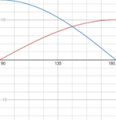Induced voltage is the blue wave form and current is the red waveform

At this point only half the cycle has been completed. Now, the current begins to descend from its maximum point. Although the magnitude of the current is decreasing, its slope is actually increasing, albeit negatively. The induced voltage still wants to oppose this rate of change. Given, that the current is still in the positive part of the graph, the voltage must now switch polarity in order to oppose the increasing rate of change of the current. That is why the voltage descends from zero into the negative part of the graph, while the current is decreasing in magnitude but increasing in negative slope. It needs to oppose and push against this increasing rate of change. Is this correct?

When the current reaches the horizontal axis, it is at its steepest slope (again negative). This means that the induced voltage must be maximally opposing the current, and therefore must be at a minimum or of a negative polarity (in the negative part of the graph) in order to oppose the changing current just before it reaches zero.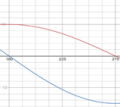Induced voltage is the blue wave form and current is the red waveform

I’m still confused about this part. What happens here? Does the current literally change directions when it hits zero or the horizontal axis? I’ve reasoned it must because after this point, the slope of the current is now decreasing again, which means that the rate of change is decreasing. The induced voltage wants to oppose this decreasing rate, and therefore will push with the current to try and maintain it at its previous instantaneous level. Is this correct?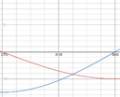Induced voltage is the blue wave form and current is the red waveform

This also means that the voltage and current must be of the same polarity so that the voltage can push the current. This explains why both the voltage and the current are in the negative part of the graph. As the current’s rate of change slows and the current reaches a minimum (maximum negative magnitude), the voltage again has less and less to oppose, and therefore decreases to zero. At this point 1 cycle of the function has been completed.

I don’t know if I can break the cycle down like I did. I’m wondering if most of this is correct, or if I’m completely wrong.

#### Jony130

Joined Feb 17, 2009
5,445
First, you must realize is that the "graph" you are trying to analyzes shows the situation in steady-state condition (the inductor was ON to the AC voltage source a very long time ago), not the transient condition (at Turn-ON time).

Also, do you know that the derivative of an of the Sine wave is a Cosine (shifted by 90 degrees sine wave)?

This equation also indicates that inductance voltage depends not on the current which actually flows through the inductance, but on its rate of change.
This means that to produce the voltage across an inductance, the applied current must change. If the current is kept constant, no voltage will be induced, no matter how large the current.

And to be able to see what is going on in AC circuit I made this "graph":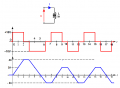Where the ideal inductor was connected directly across the "AC square waveform".
This example is easer to analysis because we can use this equation :

VL = L* ΔI/Δt

and

ΔI = VL/L *Δt

So we do not need any calculus.

If we plot the current and the voltage on the same graph we get this: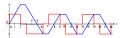At the beginning (at time 0+) we apply +10V across the inductor by doing this we are attempting to cause a sudden change in the current.
The induced voltage now steps in to try to keep the current down to its initial value (0A) and this induced voltage must be exactly equal to the applied voltage (+10V) and current start to rise-up. But do you understand why the current starts to rise up at the first place?

Remember this equation VL = L* ΔI/Δt if ΔI = 0A However, that cannot be, because zero rate of change in current implies no induced voltage.
In other words, the very existence of induced voltage depends on the fact that current changes, and it must change.We have a negative feedback mechanism here. If the current changes to fast (fast rate of change) the induced voltage will be larger than the applied voltage and this will reduce the rate of change so that this is true ΔI = VL/L *Δt = 10V/5H *1s = 2A per second. Or if the rate of change is too small then the induced voltage will be lower than the applied voltage and the current will increases the rate of change to sustain ΔI = VL/L *Δt = 10V/5H *1s = 2A per second.
Try to think about it yourself.

And in this "phase" (form t = 0s to t = 2s ) inductor stores the energy in form of a magnetic field. We can say that the inductor is in the charging phase.

At time = 3s the applied voltage change his value from +10V to -10V hence the current in the inductor starts to ramps down with rate ΔI = VL/L *Δt = 2A/s. And the inductor will start to released the stored energy and this energy is returned to the rest of the circuit when the current through the inductor is ramped down (magnetic field collapses). So, we have a discharging phase. And exactly at time t = 5s the inductor current is equal t0 0A (the inductor is fully discharged) all stored energy in form of a magnetic field was released.

But the applied voltage is still present (-10V) therefore the current will start to ramp up but this time in the opposite direction.
And again during this phase, the inductor will start to store the energy in form of a magnetic field (charging phase in the opposite direction).
And this charging phase will end at t = 6s when Vs = 0V.

From time t = 7s to t = 8s we have a discharging phase and this phase will end at t = 8s but similar as we had before, because of the fact that the applied voltage is still present the inductor current must change hence the current will continue to flow in the opposite direction (charging phase from t = 8s to t = 9s). And everything repeats itself.

I hope you now see why at AC the inductor current is zero but the voltage is not. Or maybe you did not understand anything and I make it even worse?

•BR-549